Geometry

# Tangent to Circles - Problem Solving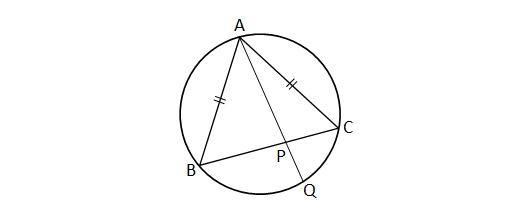As shown in the above diagram, triangle $$ABC$$ inscribed in a circle is an isosceles triangle with $$\lvert{\overline{AB}}\rvert=\lvert{\overline{AC}}\rvert,$$ where $$\lvert{\overline{AB}}\rvert$$ denotes the length of $${\overline{AB}}.$$ Given the following two lengths: $\lvert{\overline{AP}}\rvert=11 \text{ and } \lvert{\overline{PQ}}\rvert=5,$ what is $${\lvert\overline{AB}\rvert}^2?$$

Note: The above diagram is not drawn to scale.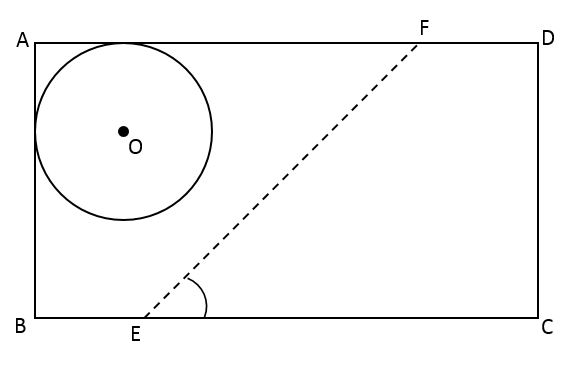The above diagram illustrates a $$17$$ meters by $$10$$ meters rectangular farmland $$ABCD.$$ The farmland contains a circular well $$O$$ with radius $$4$$ meters at its upper left corner, which is tangent to $$\overline{AB}$$ and $$\overline{AD}.$$ If the farmer wants to build a straight fence dividing the land into two areas in the following way, what should be the length of $$\overline{FD}$$ (in meters):

• $$\angle FEC = 45 ^\circ$$
• the well goes to the left of the fence
• make the area of $$FECD$$ as large as possible?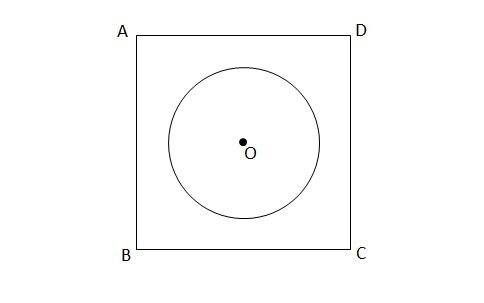$$\square ABCD$$ in the above diagram is a square-shaped park with side length $$30\sqrt{2}$$ meters. If there is a circular fountain with radius $$15$$ meters at the center of the park, what is the length (in meters) of the shortest path from $$A$$ to $$C$$ that does not pass through the fountain?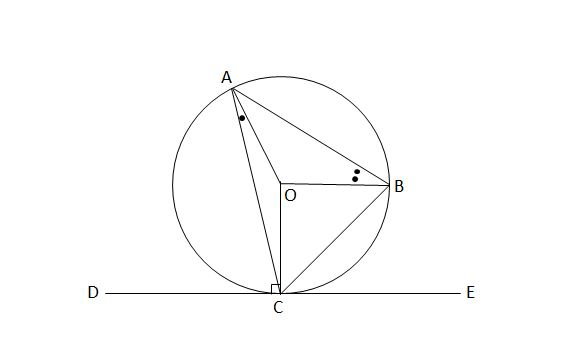$$\overline{DE}$$ is tangent to circle $$O$$ at point $$C,$$ and points $$A$$ and $$B$$ lie on circle $$O.$$ If $$\angle ACD=79 ^\circ$$ and $$\angle OBA=2\angle OAC,$$ what is the measure (in degrees) of $$\angle OCB?$$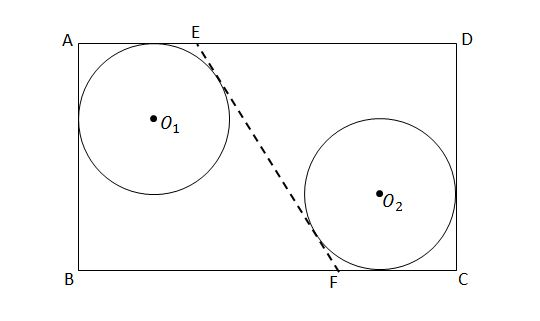You have a piece of rectangular land $$ABCD$$ that measures $$30$$ meters by $$25$$ meters. There are two wells, one at the upper left corner and another at the lower right corner, with the same radius. Both wells are tangent to the borders of the land. When you put a fence $$EF$$ that is tangent to both wells, as shown in the above diagram, you find $$\angle EFB=60^\circ.$$ What is the radius of the two wells?

×